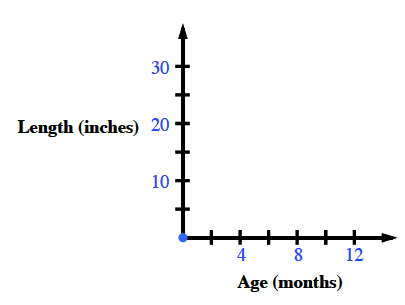### Home > MC1 > Chapter 5 > Lesson 5.2.1 > Problem5-44

5-44.

Julia collected the data in the table below about the length of her new baby sister as she grew. On graph paper, draw a pair of axes, set up a scale, and graph Julia's data.

Age (months)

Length (inches)

$0$

$19$

$1$

$20$

$4$

$23$

$6$

$25$

$7$

$26$

$9$

$28$

$12$

$31$

It would be useful to label your horizontal axis ($x$) ''Age'' and the vertical axis ($y$) ''Length.'' How do you think you should scale them?

Maybe your axes look something like the figure below? If you have drawn your axes, it is time to plot Julia's points!Your points should be written $\left(x, y\right)$ or (Age, Length).